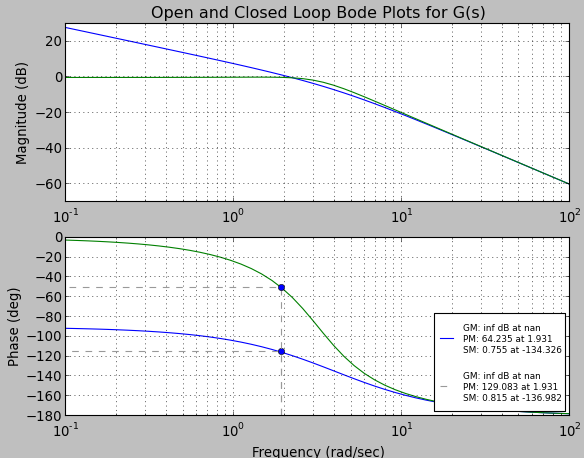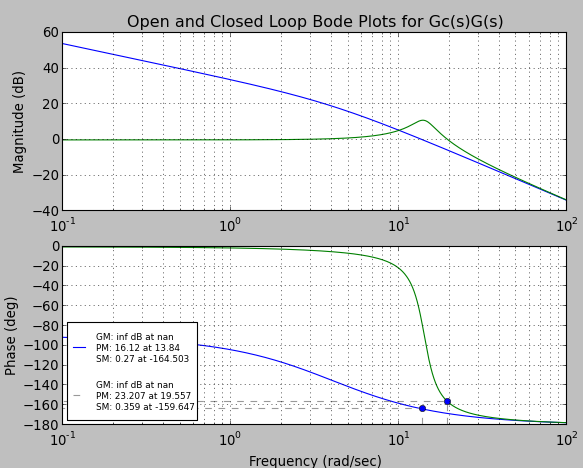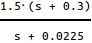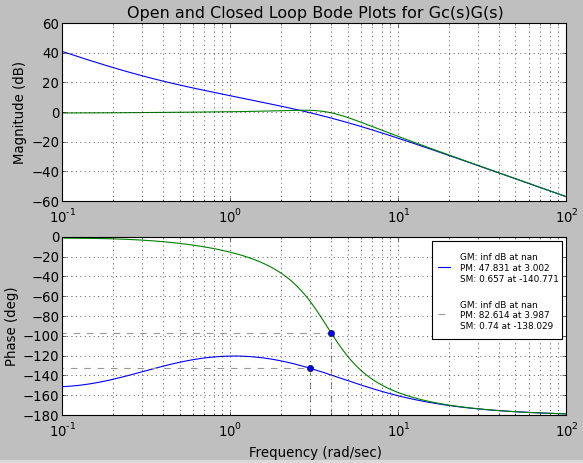### Control Systems in Python - Phase Lag Compensator Design

Here's a problem I had to do for homework. Some of the equation formatting didn't copy over, sorry...

Given an system G(s) = 10/(s(s+4)) and it’s open loop bode plots, design an appropriate compensator so that the closed loop phase margin is at least 45o and the velocity constant is 50.The bode plot shows that the PM for the open loop system is 64.2o at ω = 1.931rad/s, at the same frequency, we see that the P.M. of the closed loop system is 129o. The velocity constant indicates the steady state error to a ramp input must be ess =1/Kv = 1/50 = 0.02.  First, the velocity constant of the uncompensated system is:

Kv=limit as s->0 of sG(s) =limit as s->0 of s10/s(s+4)=10/4=2.5

since we want Kv  50, the compensator must have a gain of at least 50/2.5 = 20.

If our compensator Gc(s) = Kc = 20, we get the following new responses by simply shifting the open loop bode up by about 26dB. Thus the new open loop 0dB crossing is at  ω = 13.83 rad/s and the open and closed loop phase margins are 16.12o and  23.2o respectively.However since the specification states that the closed loop phase margin be at least 45o, this simple controller will not work.

Since we are trying to adjust the steady state error of the system instead of transient response, a phase-lag controller of the form Gc(s) = K(s+z)/(s+p) will be used. Following the phase lag design procedure for bode plots, the controller gain must be 20 or more, thus we have the condition that:
(1) Kz/p = 20

The desired crossover point will give a 45o phase margin, and the controller will add about  5o and decrease the gain by 10log(20)   13dB. So i’ll pick the frequency where the open loop phase is about 130o, at around ωc = 3 rad/s.

Then z =  ωc/10 = 0.3 and p becomes

p=0.3*20/|G(3j)|= 0.0225.
then using (1) K becomes,  20*0.0225/0.3=1.5.  The controller is then:
Gc(s) =the response then becomes:which shows that the P.M. of the open loop system is 47.8o and the P.M. of the closed loop system is 82.6o which is well above the desired amount of 45o

Recalculating the velocity constant with the compensator we get:

Kv=limit as s->0 of sGc(s)G(s)=limit as s->0 of s*1.5(s+0.3)/(s+0.0225) 10/s(s+4) =0.45*10/(0.0225*0.4)=50

which is the desired value.

### A look at Chaquopy the Python SDK for Android

I recently heard about chaquopy - A "Python SDK for Android" and was really excited. There's already a demo app on the Play store for python 2 and python 3. As the author of enaml-native , I had to take a look to see how others are trying to tackle the python on android challenge.  So here's a short review and discussion of my initial thoughts on chaquopy. Demo I encourage you to try out the demo yourself to get a feel for it, but here's a short demo of what it does. Initial thoughts. It starts reasonably fast (about 3-4 seconds on my phone).  There's a noticeable delay when starting the python activity on my phone (much smoother on the emulator), but other than that the widgets look great and interaction is smooth! The apk is 11.54 MB and the app is only 18 MB installed (21MB if you include the cache), which is very good for bundling python! Also, the build process was a piece of cake!  I cloned the repo, opened in android studio, pressed play,

### Kivy vs React-Native for building cross platform mobile apps

I've built three apps now using Kivy and one with React-Native, just wanted to share my thoughts on both. Just a warning, I am strongly biased towards python and this is all based on opinion and experience and is thus worth what you pay for it. I don't claim to be an expert in either of these, just have worked with each for several months.  If something is incorrect I'd love to hear advice. Kivy Demo of one of the apps Pros: Nice to be able to run natively on the desktop WITHOUT a simulator Python is easy to work with Use (almost) any python library Very easy to create custom widgets Kivy properties and data binding just work. Way nicer than React's "state" / flux / redux whatever you want to call it (stupid?).  Native interfaces (pyjnius) and (pyobjc) Runs and feels pretty smooth Cons: Default widget toolkit looks like Android 4.4. Requiring you use your own widgets or a theming kit like KivyMD  if styling bothers you Creating dy

### Control Systems in Python - Part 1 - Bode and Step Response

I hate matlab with passion, yet sadly, nearly everyone uses it.  I'm a fan of Python and open source stuff so here's a simple article on how to do some common control systems stuff in Python. First we need to make sure the environment is setup. Install IPython (or you can use any other python shell, but a unicode supported shell is preferred) Install python-control (numpy, scipy) Install sympy These should do if your on Ubuntu/debian: sudo apt - get install python - sympy python-numpy python-scipy python-matplotlib ipython Then you need to install python control, see How to download and install python-control Intro to using Sympy Open ipython and run the following: import sympy from sympy import * sympy.init_printing() s = Symbol('s') Now we can do things like define transfer functions using the symbolic variable s. We can expand the bottom using the .simplify() method and we can do something more complex like... which is really nice because it